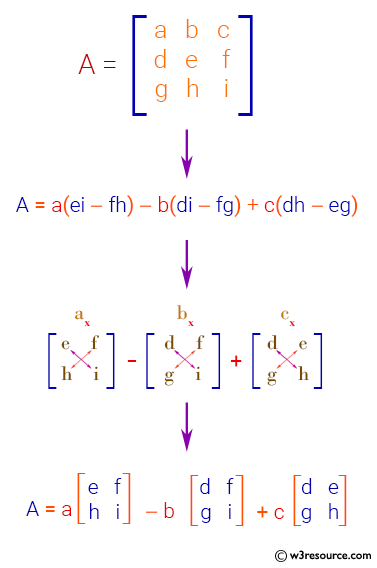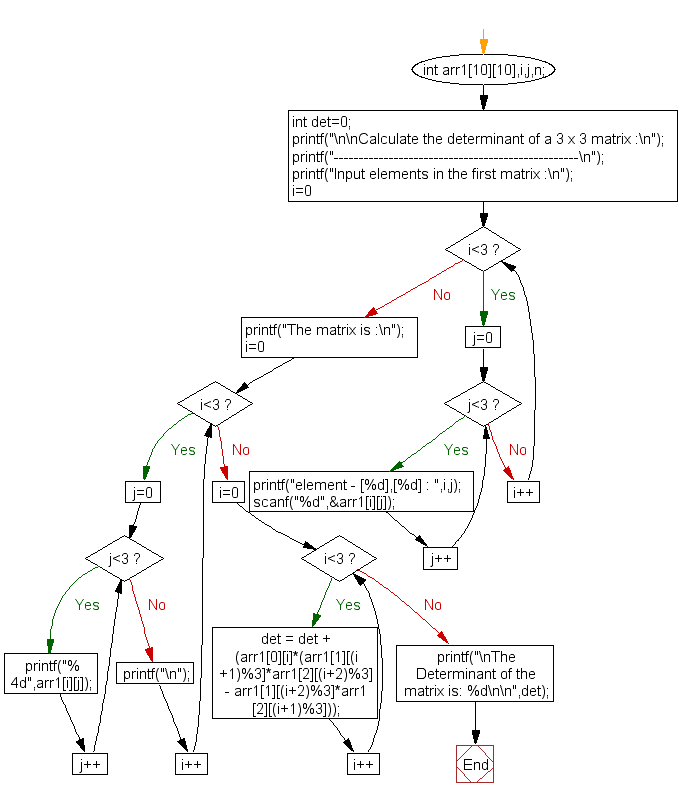﻿ C exercises: Calculate the determinant of a 3 x 3 matrix - w3resource# C Exercises: Calculate the determinant of a 3 x 3 matrix

## C Array: Exercise-28 with Solution

Write a program in C to calculate determinant of a 3 x 3 matrix.

Pictorial Presentation:Sample Solution:

C Code:

``````#include <stdio.h>

void main()
{
int arr1,i,j,n;
int det=0;

printf("\n\nCalculate the determinant of a 3 x 3 matrix :\n");
printf("-------------------------------------------------\n");

printf("Input elements in the first matrix :\n");
for(i=0;i<3;i++)
{
for(j=0;j<3;j++)
{
printf("element - [%d],[%d] : ",i,j);
scanf("%d",&arr1[i][j]);
}
}
printf("The matrix is :\n");
for(i=0;i<3;i++)
{
for(j=0;j<3 ;j++)
printf("% 4d",arr1[i][j]);
printf("\n");
}

for(i=0;i<3;i++)
det = det + (arr1[i]*(arr1[(i+1)%3]*arr1[(i+2)%3] - arr1[(i+2)%3]*arr1[(i+1)%3]));

printf("\nThe Determinant of the matrix is: %d\n\n",det);
}
```
```

Sample Output:

```Calculate the determinant of a 3 x 3 matrix :
-------------------------------------------------
Input elements in the first matrix :
element - , : 1
element - , : 0
element - , : -1
element - , : 0
element - , : 0
element - , : 1
element - , : -1
element - , : -1
element - , : 0
The matrix is :
1   0  -1
0   0   1
-1  -1   0

The Determinant of the matrix is: 1
```

Flowchart:C Programming Code Editor:

Improve this sample solution and post your code through Disqus.

What is the difficulty level of this exercise?

﻿